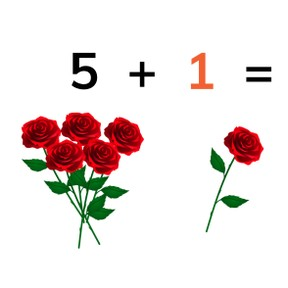Addition to 10 with 1 or 2 more

# Addition to 10 with 1 or 2 more

Addition to 10 with 1 or 28,000 schools use Gynzy92,000 teachers use Gynzy1,600,000 students use Gynzy

## General

Students learn to add to 10, by adding 1 or 2 to a number.

## Relevance

It is important to be able to add 1 or 2 to a number, so you know how much you have if you add 1 or 2.

## Introduction

The interactive whiteboard shows different numbers of books, pens, and scissors. The students must count the amounts that are there. You can erase the grey blocks to show the answers.

## Development

Using the example on the interactive whiteboard, you can explain addition by counting one more. Count the amount of flags, and then drag one more flag, and count the new total. Next you can show addition by using the apples- counting the amount, and the ones that are being added, and giving the total. By doing so you encourage students to count how many they already have, and then to count on with the new objects, to come to the total. To check that students can count by adding one or two more, you can count the amounts on the interactive whiteboard and make sure that they always count one or two more. Eventually you show them how to add without images, and only using numbers. Emphasize that the first number is how much you already have, and the second tells us how many more are being added. You count the total by counting one or two more.

To check that students understand addition by counting one or two more, you can ask the following questions:
- What does + mean?
- What do you do if you want to know how much 3 + 1 is?
- What do you do if you want to know how much 5 + 2 is?

## Guided practice

Students first practice with counting amounts. They then practice addition by counting one or two more.

## Closing

Discuss with students that it is important to be able to add to 10 by counting on, so you know how many objects you have if 1 or 2 more are added. Next the students count the numbers in the flags by counting one more. They must choose from three given answers. To check the answer, drag the flag. If the answer is correct, a checkmark is revealed. If the answer is incorrect, a red x is revealed. Students repeat this but with counting two more.

## Teaching tips

If students have difficulty with this goal, students can use blocks or other manipulatives, so they can manually move one or two more to a given number.

### The online teaching platform for interactive whiteboards and displays in schools

• Save time building lessons

• Manage the classroom more efficiently

• Increase student engagement